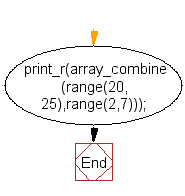﻿ PHP Array Exercise: Create a range of specified arrays - w3resource# PHP Array Exercises : Create a range of specified arrays

## PHP Array: Exercise-59 with Solution

Write a PHP program to create a range like the following array.

Array
(
 => 2
 => 3
 => 4
 => 5
 => 6
 => 7
)

Sample Solution:

PHP Code:

``````<?php
print_r(array_combine(range(20, 25),range(2,7)));
?>
```
```

Sample Output:

```Array
(
 => 2
 => 3
 => 4
 => 5
 => 6
 => 7
)
```

Flowchart:PHP Code Editor:

What is the difficulty level of this exercise?

﻿

## PHP: Tips of the Day

PHP: Extract numbers from a string

```\$str = 'In My Cart : 11 12 items';
preg_match_all('!\d+!', \$str, \$matches);
print_r(\$matches);
```